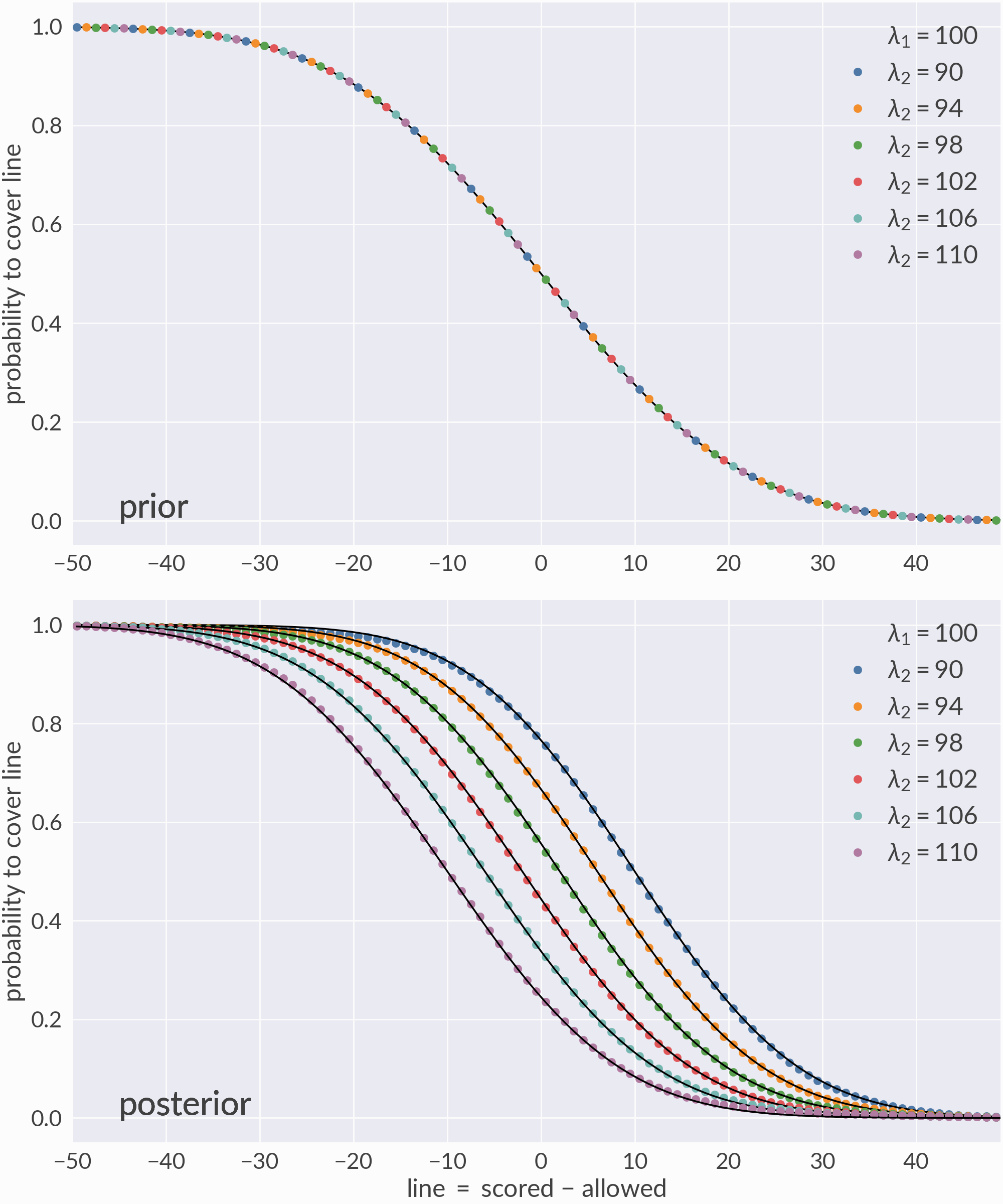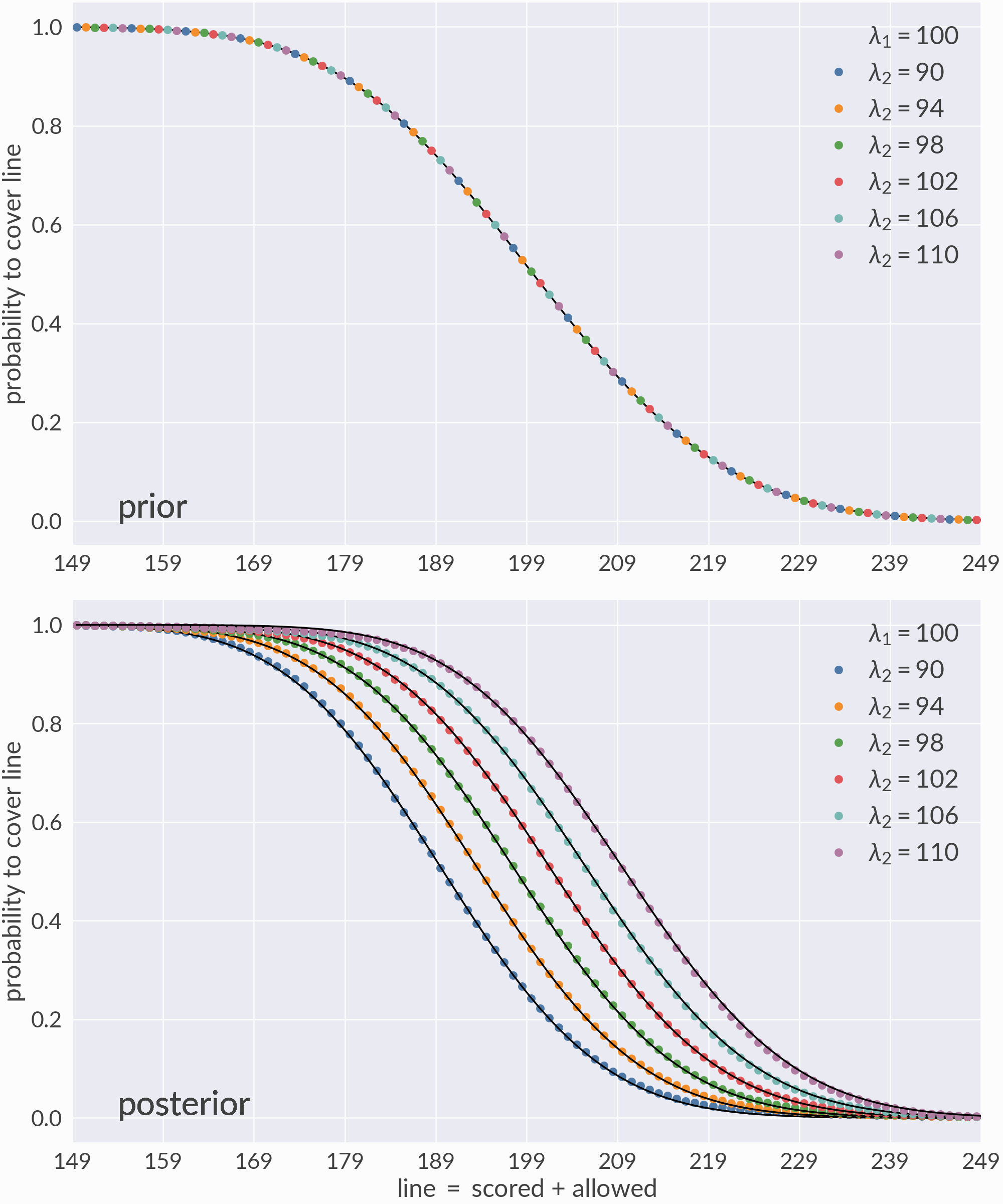# Tests¶

melo has a standard set of unit tests, whose current CI status is:The unit tests do not check the physical accuracy of the model which is difficult to verify automatically. Rather, this page shows a number of visual tests which can be used to access the model manually.

## Toy model¶

Consider a fictitious “sports league” of eight teams. Each team samples points from a Poisson distribution $$X_\text{team} \sim \text{Pois}(\lambda_\text{team})$$ where $$\lambda_\text{team}$$ is one of eight numbers

$\lambda_\text{team} \in \{90, 94, 98, 100, 102, 106, 110\}$

specifying the team’s mean expected score. I simulate a series of games between the teams by sampling pairs $$(\lambda_\text{team1}, \lambda_\text{team2})$$ with replacement from the above values. Then, for each game and team, I sample a Poisson number and record the result, producing a tuple

$(\text{time}, \text{team1}, \text{score1}, \text{team2}, \text{score2}),$

where time is a np.datetime64 object recording the time of the comparison, $$\text{team1}$$ and $$\text{team2}$$ are strings labeling each team by their $$\lambda$$ values, and $$\text{score1}$$ and $$\text{score2}$$ are random integers. This process is repeated $$\mathcal{O}(10^6)$$ times to accumulate a large number of games.

I then calculate the score difference or $$\text{spread} \equiv \text{score1} - \text{score2}$$ for each game to form a list of comparisons $$(\text{time}, \text{team1}, \text{team2}, \text{spread})$$ and use these comparisons to train the margin-dependent Elo model:

lines = np.arange(-49.5, 50.5)
model = Melo(1e-4, lines=lines, commutes=False)

Now that the model is trained, I can predict the probability that various matchups cover each value of the line, i.e. $$P(\text{spread} > \text{line})$$. Since the underlying distributions are known, I can validate these predictions using their analytic results.